Google documents and spreadsheets offer a great way of creating spreadsheets and documents that can be used for collaboration between various people. Launched in 2006, Google spreadsheets have gained in popularity as a way to share and collaborate on spreadsheet data.

Google spreadsheets have come a long way since their launch, and the application now offers a comprehensive list of functions and formulas to allow for data analysis and manipulation. The Google Spreadsheets Step by Step course offers you over 66 lectures and four hours of content that will show you how to get the most out of this free spreadsheet software application.

The following article will show you how to use some of the Google spreadsheet functions in your own Google spreadsheets. A lot of the functions are very similar to the functions and formulas found in Excel so if you are familiar with functions and formulas in Excel, then the transition to Google spreadsheets should be a painless transition. Excel Formulas & Functions – In depth is a great resource that will teach you how to use the functions and formulas in Excel and most of these functions are applied in exactly the same way in Google Spreadsheets.

To insert a function into your Google Spreadsheet, you need to begin by selecting a target cell for the function or formula. The target cell is the cell where you want to answer to appear. Once you have selected the target cell, you can insert the function or formula by typing the name of the formula into the cell directly. You can also select the formula from the “Insert”, “Function” menu available in Google: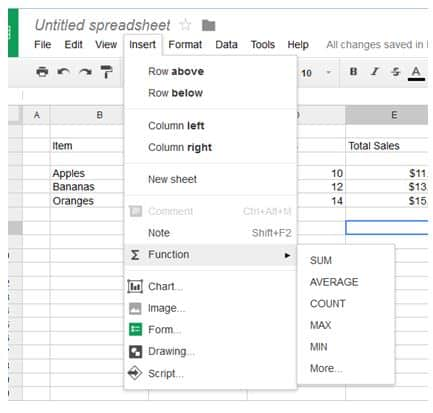There are four functions available from the “Insert”, “Function” list. These include the SUM function, the AVERAGE function, the COUNT function, the MAX function and the MIN function.

Let’s examine how you can use some of these functions in your spreadsheet.

## The SUM and SUBTOTAL functions in Google Spreadsheets

The SUM function allows you to simply and quickly add up numbers within various cells or within a range of cells. The SUBTOTAL function allows you to add up values without including other SUBTOTALS included within the range.

To use the SUM function, click your target cell, then click “Insert”, “Function” and select the SUM function from the menu. The Google Spreadsheet application provides a description of how each function works and how to set the parameters for each function: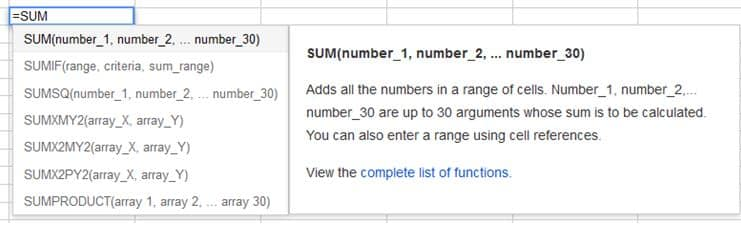Here is an example of how to use the SUM function in a Google spreadsheet. You can enter the function into the target cell directly or ou can select the target cell and then ype the formula into the Fx cell at the top of your worksheet: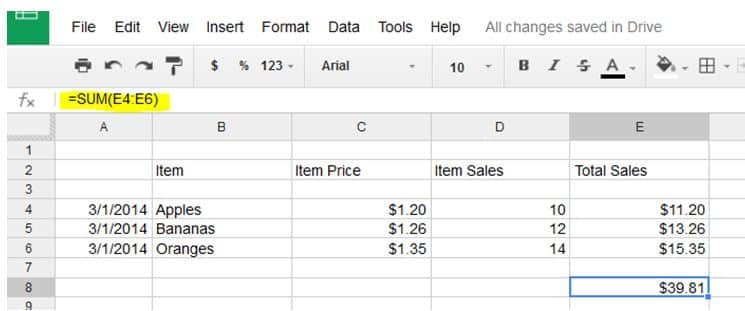The highlighted cell indicates the SUM function used to create a SUM of all the sales we made for the 1st of March.

If we add sales for the 2nd of March and then create a SUM for the sales on the 2nd of March, then the spreadsheet will look like this: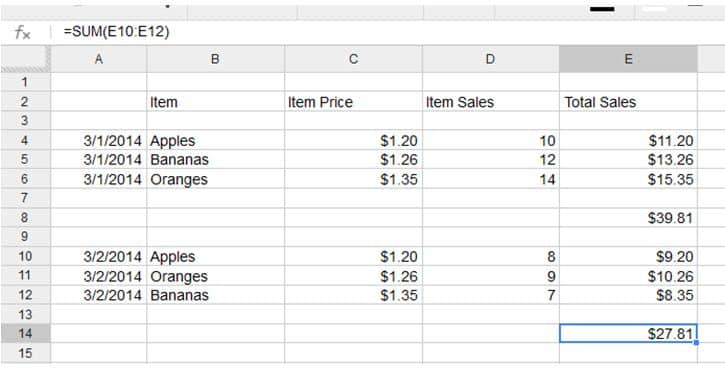If we now use the SUM function to calculate the total sales to date, our spreadsheet will look like this: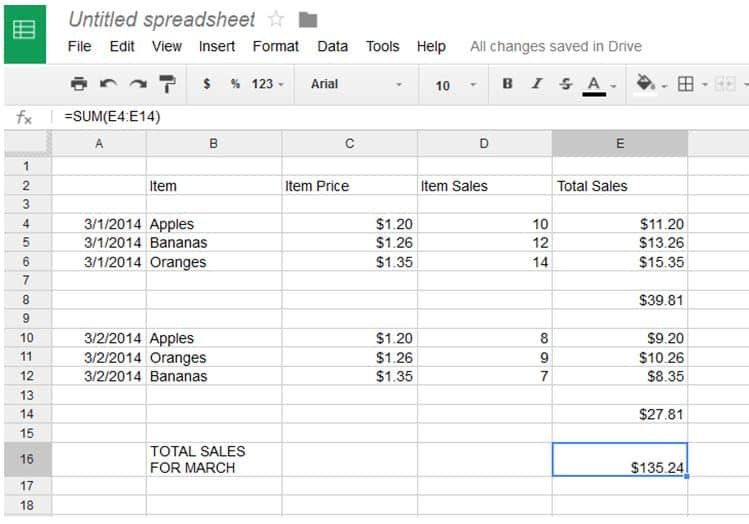This total is incorrect. The SUM function adds the values in the columns above, so it has added the two SUM values at the end of each day to the total sales. To calculate the sales without including the other SUM values, it is better to use the SUBTOTAL function to calculate the daily sales and total sales.

The SUBTOTAL function in Google Spreadsheets also adds up the values in the cells or ranges you select. The SUBTOTAL function is really helpful where you need to create subtotals within a range and you then need to calculate the totals for the entire range.

Here is an updated spreadsheet using SUBTOTALS for each day instead of using the SUM function: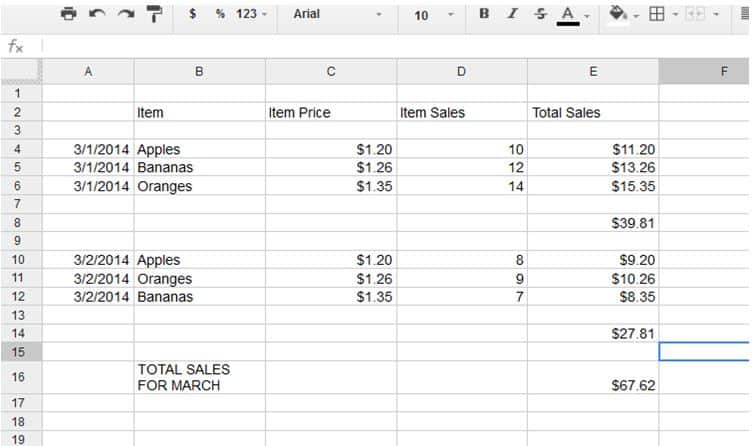The SUM and SUBTOTAL functions in Google Spreadsheets is very similar to the SUM and SUBTOTAL functions within Excel. Excel Subtotal Function: What It Is and How It can Save You Time is an excellent resource that will show you how to use the SUM and SUBTOTAL functions in Excel.

## The AVERAGE function in Google Spreadsheets

The AVERAGE function in Google Spreadsheets is used to calculate the average value of a range or selection of cells.

To calculate the AVERAGE sales per day using our example spreadsheet, you can type the following into the target cell:

=AVERAGE(E4:E12)

The result will look like this: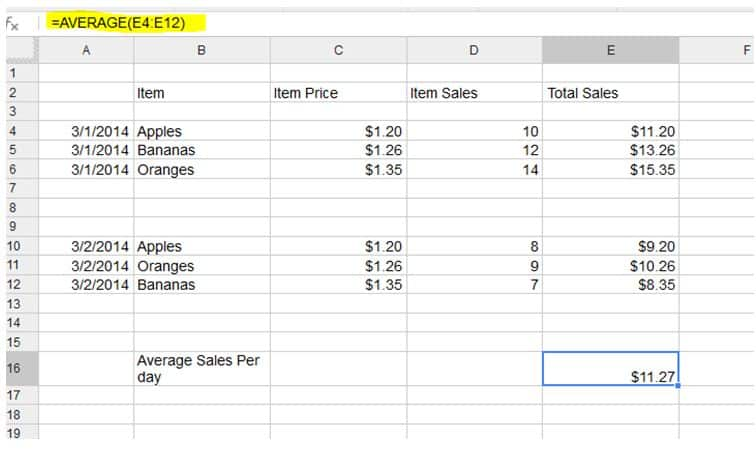The AVERAGE function in Google Spreadsheets works like the AVERAGE function in Excel. For an excellent article on how to use the AVERAGE function in Excel, read the Average Function in Excel: Using AVERAGE in Microsoft Excel article available from the Udemy blog.

## The MAX function in Google Spreadsheets

The MAX function in Google Spreadsheets allows you to quickly find the largest value in a given set of cells or data range. If we wanted to find the largest sale we’d made using our example spreadsheet, then we could use the MAX function to find the largest sale. To use the MAX function in our Google spreadsheet we could type the following into our target cell:

=MAX(E4:E12)

The resultant spreadsheet would look like this: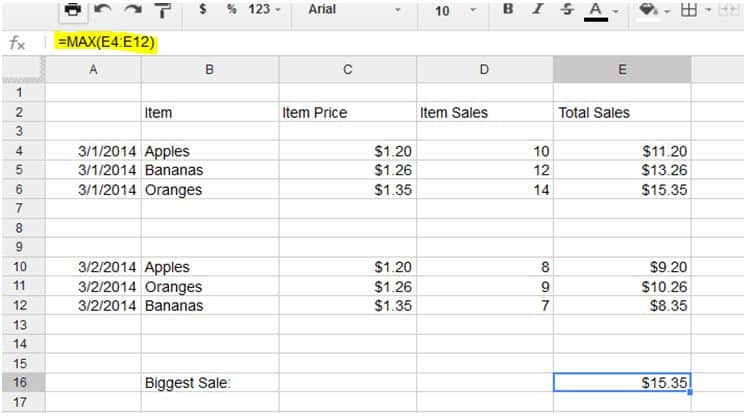## The MIN function in Google Spreadsheets

The MIN function in Google Spreadsheets allows you to quickly search for the smallest value within a given data range or range of cells. To use the function in our example worksheet, you can select Insert Function from the menu and then select the “MIN” function, or you can type the following formula into the target cell:

=MIN(E4:E12)

The result of the formula will look like this: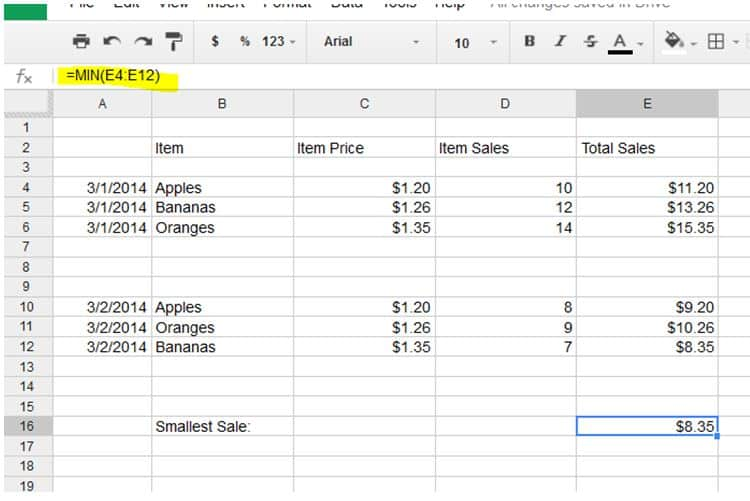As mentioned above, most of the Google Spreadsheet functions work in exactly the same as the functions available from Excel.

The Microsoft Excel – Advanced Formulas And Functions course from Udemy is a great way to learn how to apply the functions and formulas available in Excel. For most functions, if you can apply them in Excel then you will be able to apply them in Google Spreadsheets too using the same parameters and formulas.

Page Last Updated: March 2014

### Featured course

Google Sheets Basics: An Introduction to Google Sheets

Last Updated May 2022

• 4 total hours
• 41 lectures
• Beginner Level
5 (1)

Get started with Google Sheets with this comprehensive course for absolute beginners | By Simon Sez IT

## Empower your team. Lead the industry.

Get a subscription to a library of online courses and digital learning tools for your organization with Udemy Business.